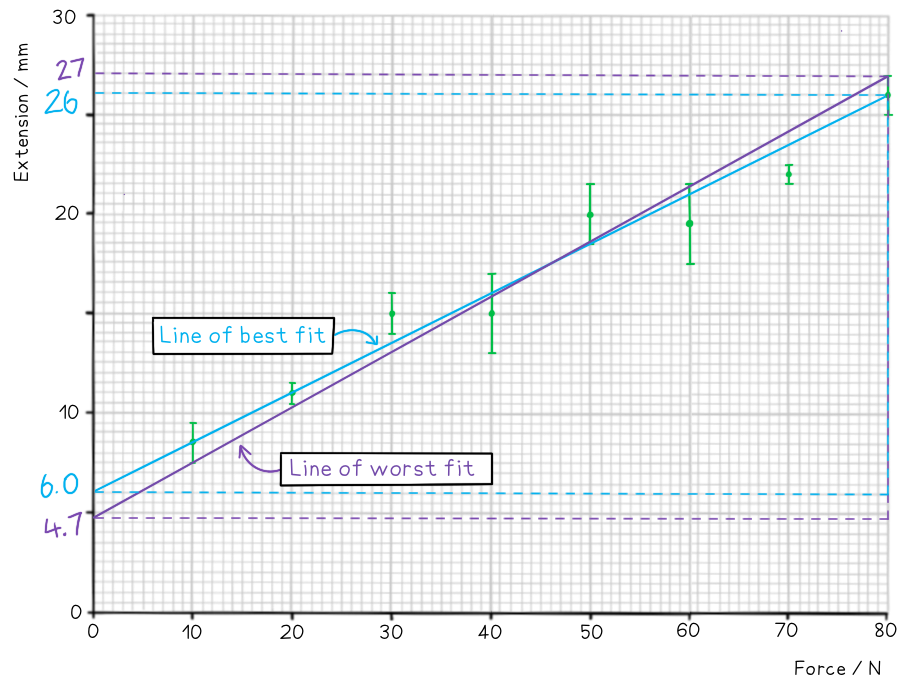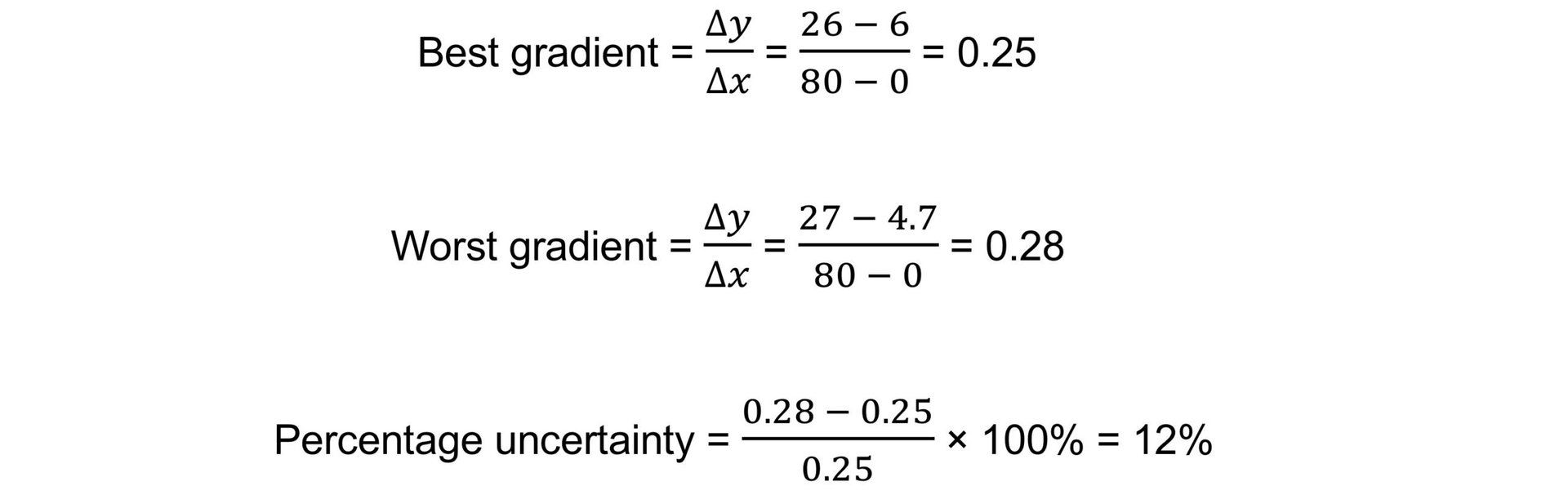# IB DP Physics: SL复习笔记1.2.3 Determining Uncertainties from Graphs

### Error Bars

• The uncertainty in a measurement can be shown on a graph as an error bar
• This bar is drawn above and below the point (or from side to side) and shows the uncertainty in that measurement
• Error bars are plotted on graphs to show the absolute uncertainty of values plotted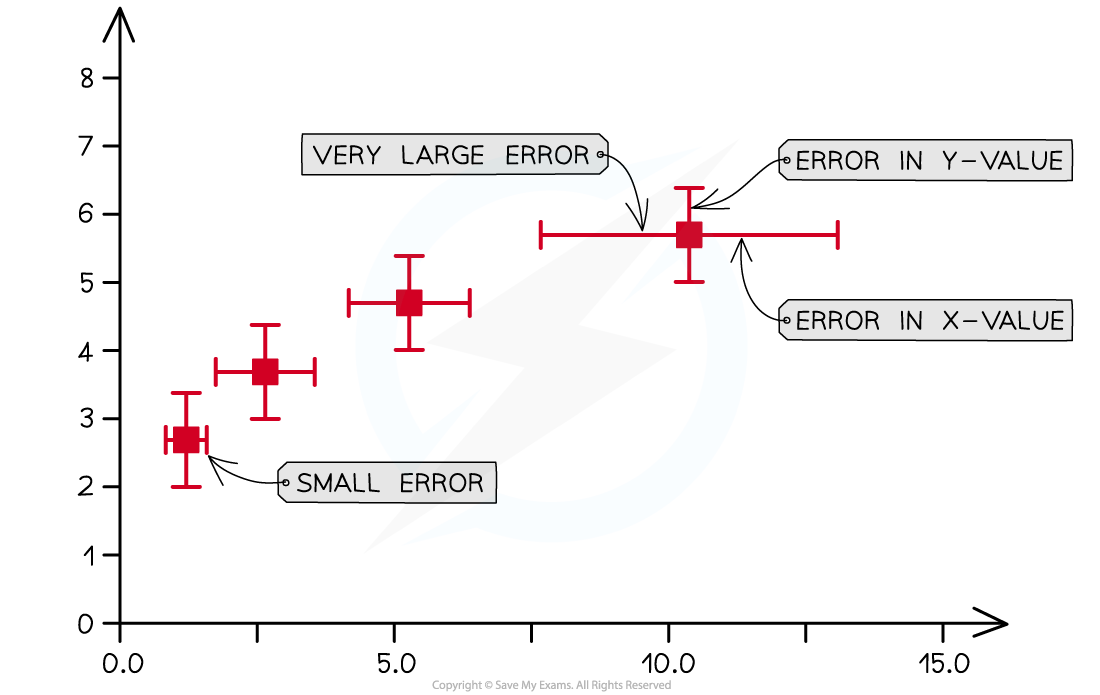Representing error bars on a graph

#### Exam Tip

A common misconception is that error bars need to all be the same size. In physics, this is not the case and each data point can have different error bar sizes as they have different uncertainties.

### Determining Uncertainties from Graphs

• To calculate the uncertainty in a gradient, two lines of best fit should be drawn on the graph:
• The ‘best’ line of best fit, which passes as close to the points as possible
• The ‘worst’ line of best fit, either the steepest possible or the shallowest possible line which fits within all the error bars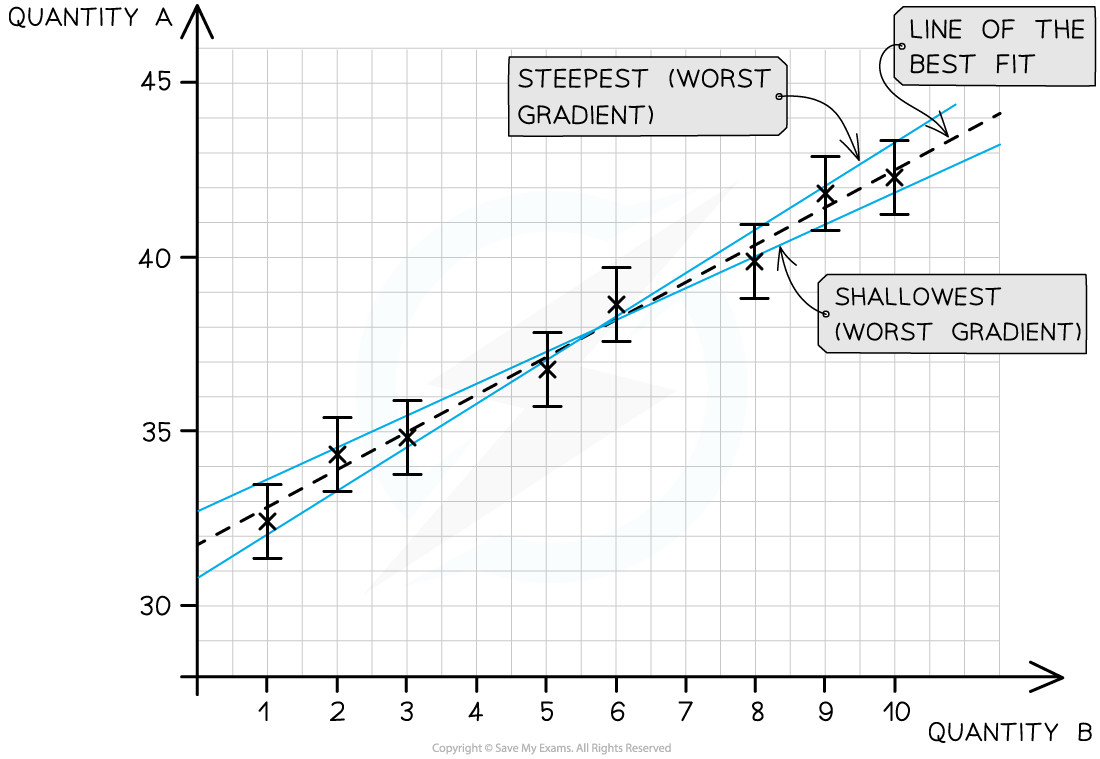The line of best fit passes as close as possible to all the points. The steepest and shallowest lines are known as the worst fit

###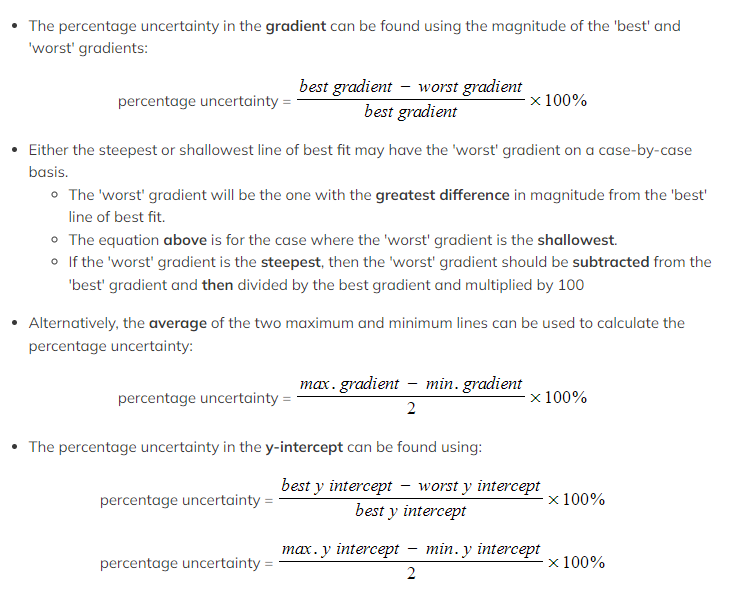Percentage Difference

• The percentage difference gives an indication of how close the experimental value achieved from an experiment is to the accepted value
• It is not a percentage uncertainty
• The percentage difference is defined by the equation: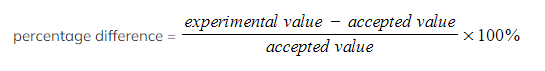• The experimental value is sometimes referred to as the 'measured' value
• The accepted value is sometimes referred to as the 'true' value
• This may be labelled on a component such as the capacitance of a capacitor or the resistance of a resistor
• Or, from a reputable source such as a peer-reviewed data booklet
• For example, the acceleration due to gravity g is known to be 9.81 m s–2. This is its accepted value
• From an experiment, the value of g may be found to be 10.35 m s–2
• Its percentage difference would therefore be 5.5 %
• The smaller the percentage difference, the more accurate the results of the experiment

#### Worked Example

On the axes provided, plot the graph for the following data and draw error bars and lines of best and worst fit.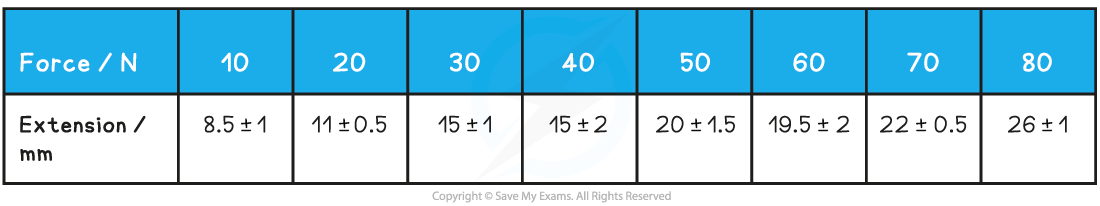Find the percentage uncertainty in the gradient from your graph.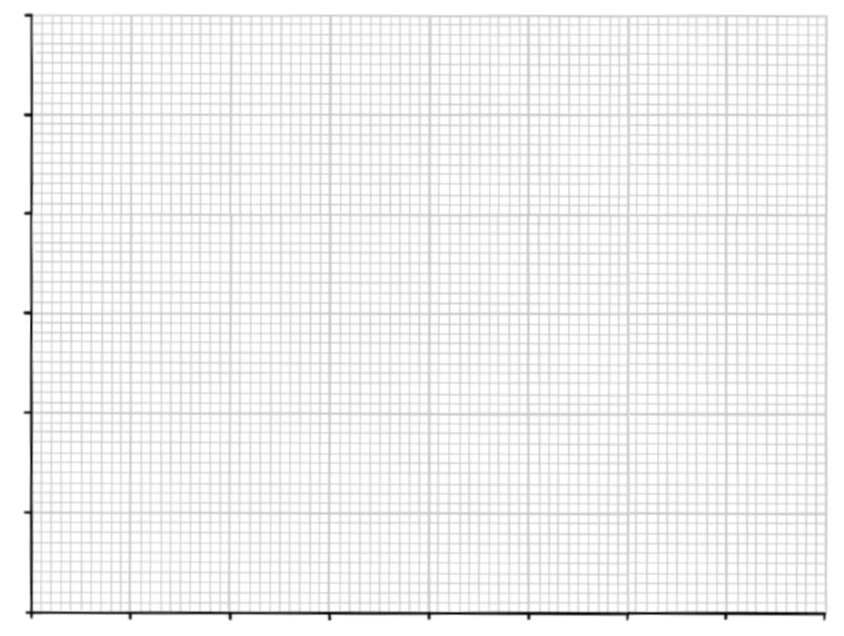Step 1: Draw sensible scales on the axes and plot the data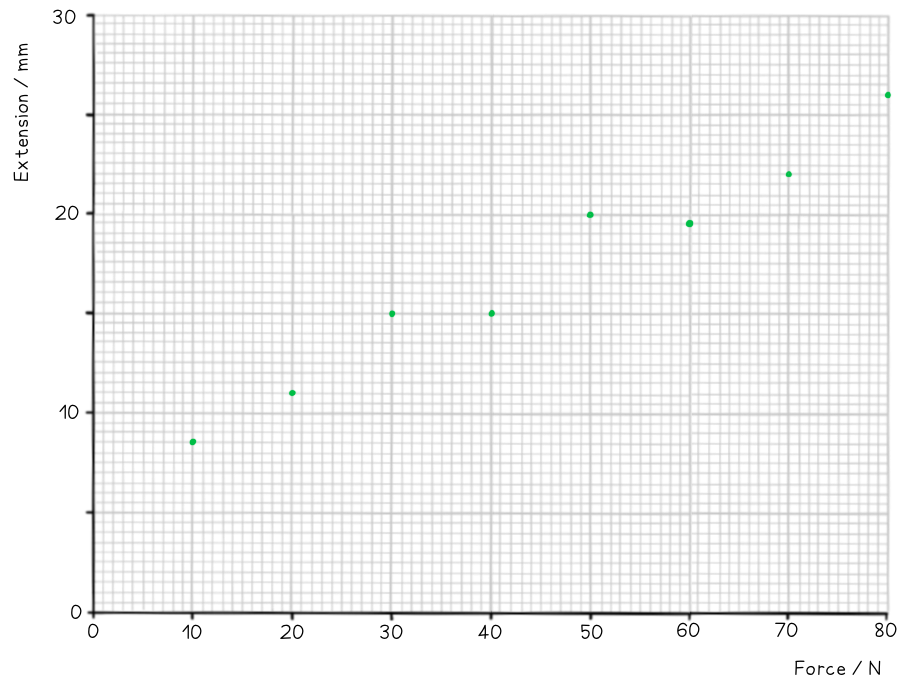Step 2: Draw the errors bars for each point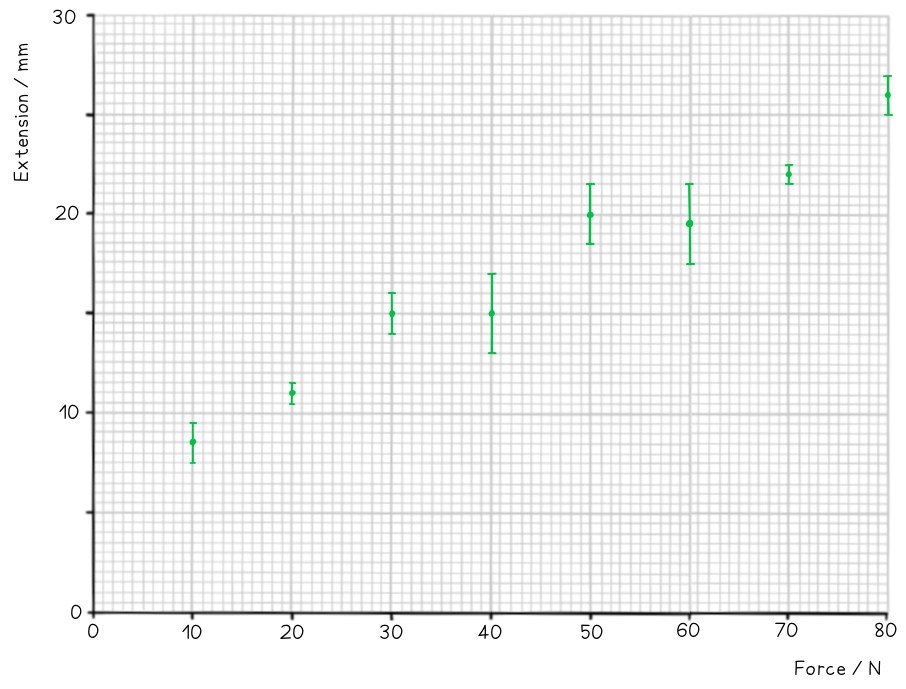Step 3: Draw the line of best fit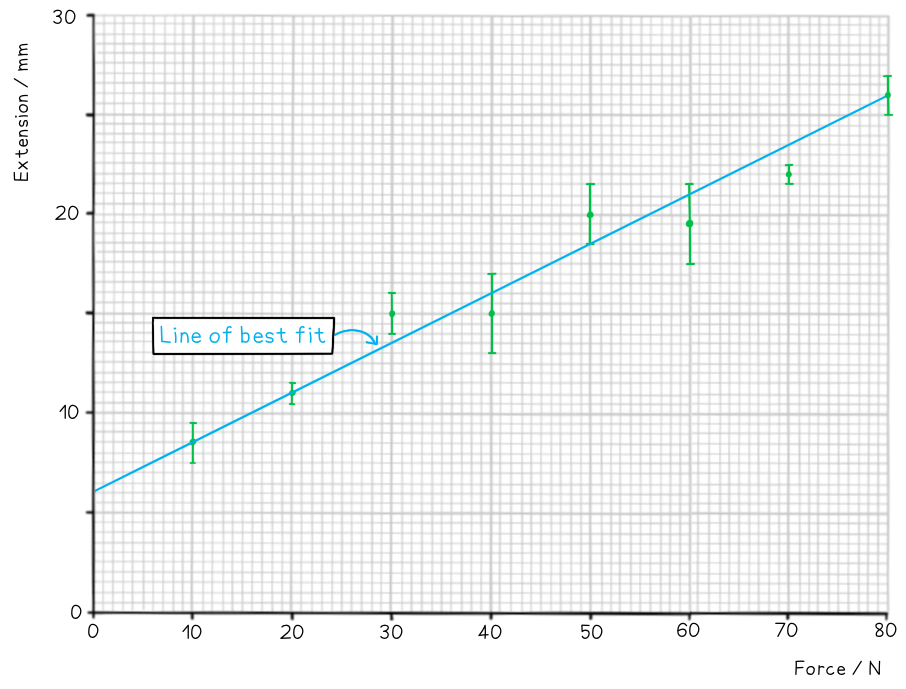Step 4: Draw the line of worst fit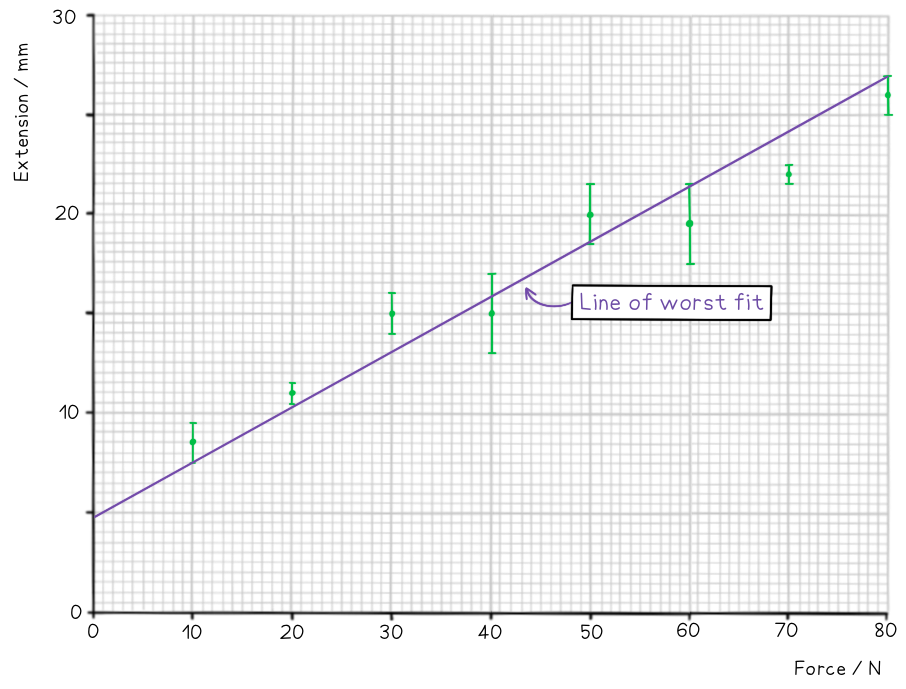Step 5: Work out the gradient of each line and calculate the percentage uncertainty